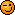> >>

# Mistake in the ACE the technical Pilot Interview

Professional Pilot Training (includes ground studies) A forum for those on the steep path to that coveted professional licence. Whether studying for the written exams, training for the flight tests or building experience here's where you can hang out.

# Mistake in the ACE the technical Pilot Interview

Join Date: Sep 2012
Location: In the sky
Age: 35
Posts: 30
Mistake in the ACE the technical Pilot Interview

Dear all,

Maybe i'm wrong but during some train in "performance and flight training" page 214 (edition 2 of Ace the technical Pilot Interview), i found a error concerning the PNR.
Indeed, in the book he is written to find the PNR you have to calculate like this :
PNR = E*H / O*H

According to bristol ground school PNR means Point of safe return (Enough fuel reserve to go back at your departure airport).
In Bristol ground school the formula is :

PNR (PSR) = E*H / O + H

E mean time, H mean GS home, O mean GS out.

Could you please check it if i'm wrong or not ?

Thanks everydobyJoin Date: Nov 2000
Location: White Waltham, Prestwick & Calgary
Age: 68
Posts: 3,847
Bristol are correct - and to prove it:

To get the simple (normal) PNR time in minutes, we start with the observation that the distances out to the PNR and back are the same, as you are trying to return to where you started. However, distance is equal to groundspeed multiplied by time, so:

O x T = H x (E-T)

Where E is the safe endurance (i.e. allowing for reserves), H the homebound groundspeed and O the outbound.

Moving on:

OT = HE - HT

OT + HT = HE

T x (O + H) = HE

E x H
O + H

All the formula does is find the ratio of the groundspeeds and divide them into the endurance, assuming normal TAS. For example, with 3 hours' endurance, and a 90 kt groundspeed outbound, with 150 home, the equation is:

180 x 150
90 + 150

27000
240

The answer is 112.5 minutes, based on a ratio of 9:15, which is actually the same as the speeds below the line without the zero.Join Date: Dec 2004
Location: A place where something is or could be located; a site.
Posts: 455
Hi Alan,

You are correct; PNR = EH / (O+H).

If that's the only error you have found in the book then you must have only just bought it?

It's full of errors.

BUT

Very useful for the types of questions you might get.Join Date: Sep 2012
Location: In the sky
Age: 35
Posts: 30
Hey EK4457

Just start to read flight planning and performance. So you confirme it's full of errors in this book ?

If it's true, we have to notice them, it's not normal.Join Date: Jan 2011
Location: England
Posts: 644
In situations like this it can be helpful to look at what the equations would yield.

In this case we are looking for the time to PNR and time is measured in hours.

Endurance is also measured in hours. Ground speed is measured in knots which are NM/hour

So in the equation we have PNR = E*H / O*H

Hours to PNR = (Hours x NM/hour ) / (NM/hour x NM/hour)

Which simplifies to give:

Hours to PNR = NM / ( NM squared x hours squared )

Dividing top and bottom by Nm gives:

Hours to PNR = 1 / (NM x hours squared )

Multiplying top and bottom by hours squared gives:

Hours to PNR = ( Hours squared / NM )

I dont know what an hours squared/NM looks like, but it is clear that hours and hours squared / NM are not the same thing. So this first equation does not yield a time in hours.

Now lets try the second equation

PNR = E*H / O + H

Hours to PNR = ( Hours x NM/Hour) / (Nm/hour + NM/hour )

This simplifies to give:

Hours to PNR = ( Hours x NM/Hour) / (Nm/hour )

Dividing top and bottom by NM/hour gives

Hours to PNR = ( Hours ) / ( 1 )

Multiplying the top and bottom by 1 gives;

Hours to PNR = Hours

So this second equation does yield an answer that is in the correct units (hours).

The process as written above looks a bit complicated, but this is because I have explained each step. But in practice it is easy provided you are reasonably good at maths. If you are not reasonably good at maths it will take a bit more effort, but the practice will do you good.You may not post new threads
You may not post replies
You may not post attachments
You may not edit your posts

BB code is On
Smilies are On
[IMG] code is On
HTML code is OffShow Printable VersionEmail this Page